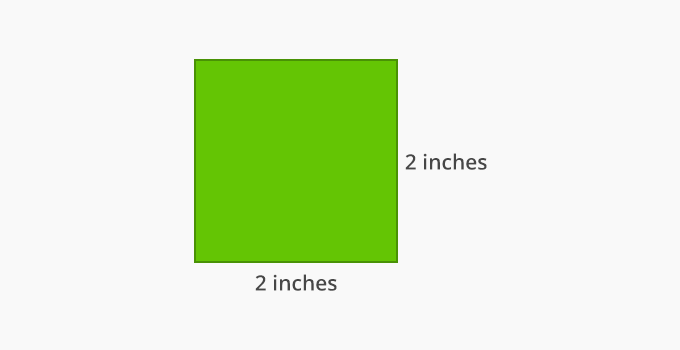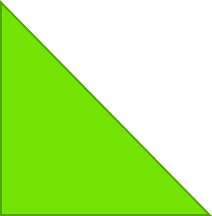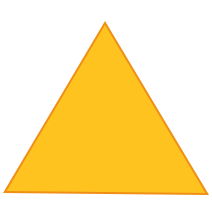# Attribute - Definition with Examples

The Complete K-5 Math Learning Program Built for Your Child

• 40 Million Kids

Loved by kids and parent worldwide

• 50,000 Schools

Trusted by teachers across schools

• Comprehensive Curriculum

Aligned to Common Core

## What is Attribute?

The term “attribute” in the context of math means the traits or the properties of a shape or an object. For example, observe the following picture of books.In the above image, the traits of the books can be listed as:

• Shape – Rectangle

• Color – Red and Blue

• Page count

• Length & Breadth in a given unit

• Weight

## Attributes of a shape

The attributes of a geometric shape define the characteristics of that shape visually and mathematically. For example, consider a “square” as shown below. The attributes of a square are:Visual Attributes Mathematical Attributes Shape has 4 sides and 4 vertices All sides are equal Opposite sides are parallel Each side measures 2 inches The angle between any two adjacent sides is 90 degree

Similarly, based on the attributes, we can determine the type of triangle:Type: Right-angle triangle The color of the triangle is Green Two sides can be equal The angle between the two sides is 90 degree Type: Equilateral triangle The color of the triangle is Yellow All 3 sides are equal The angle between the two adjacent sides is 60 degrees Type: Scalene triangle The color of the triangle is Blue All 3 sides are unequal  One of the angles between the two sides is more than 90 degrees

The unique attributes or properties of geometric shapes help us to distinguish and categorize them.

## Application

The elementary math skills in children initiate with visual observation before understanding numbers and comprehending them. Understanding the attributes of geometric figures, math expressions (such as symbols and operators) and translating them in the form of numbers is the key step achieved with finding “attributes”.

 Fun Facts Astronomers explored and named the famous star constellations after zodiac signs by finding similar attributes between them Computer programming and code writing are logic development skills which uses the functions & attributes of a programming language

## Related math vocabulary

• Properties of geometric shapes